### Home > CCA2 > Chapter 8 > Lesson 8.2.2 > Problem8-90

8-90.
1. Consider this geometric sequence: i0, i1, i2, i3, i4, i5 , …, i15. Homework Help ✎

1. You know that i0 = 1, i1 = i, and i2 = −1. Calculate the result for each term up to i15, and describe the pattern.

2. Use the pattern you found in part (a) to calculate i16, i25, i39, and i100.

3. What is i4n , where n is a positive whole number?

4. Based on your answer to part (c), simplify i4n+1, i4n+2, and i4n+3.

5. Calculate i396, i397, i398, and i399.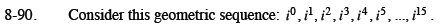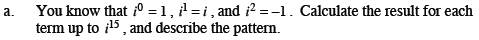i0 = 1 Can you see the pattern?
i1 = i
i2 = −1
i3 = (i)(i2) = −i
i4 = (i2)(i2) = 1
i5 = (i2)(i2)(i) = i

The pattern repeats 1, i, −1, and −i.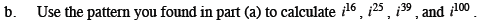16 is a multiple of 4.
25 is one more than a multiple of 4.
39 is one less than a multiple of 4.
100 is a multiple of 4.

i16 = 1
i25 = i
i39 = −i
i100 = 1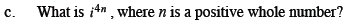4n is a multiple of 4.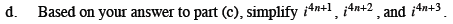Refer to (a), (b), and (c).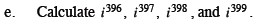i396 = i4 · 99 = 1
i397 = i4 · 99 + 1 = ?
i398 = i4 · 99 + 2 = ?
i399 = ?